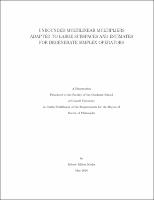## Unbounded Multilinear Multipliers Adapted To Large Subspaces And Estimates For Degenerate Simplex Operators##### Abstract
We prove in Chapter 1 that for every integer n [GREATER-THAN OR EQUAL TO] 3 the n-sublinear map n ˆ fj ([xi]j )e2[pi]ix[xi]j d[xi] nC [alpha] : (f1 , ..., fn ) [RIGHTWARDS ARROW] sup M [xi][MIDDLE DOT][alpha]>0,[xi]n <M j=1 [-]1 satisfies no Lp estimates provided [alpha] ∈ Rn satisfies [alpha]j = [alpha] + qj for some q ∈ Qn with distinct entries and [alpha] ∈ R with qj = [-][alpha] for all 1 [LESS-THAN OR EQUAL TO] j [LESS-THAN OR EQUAL TO] n. Furthermore, if [-]1 2 n [GREATER-THAN OR EQUAL TO] 5 and [alpha] ∈ Rn satisfies [alpha]j = qj + [alpha]qj for some q ∈ Qn with distinct, non-zero entires such that qj [alpha] = [-]1 for all 1 [LESS-THAN OR EQUAL TO] j [LESS-THAN OR EQUAL TO] n, it is shown that there is a symbol m : Rn [RIGHTWARDS ARROW] C adapted to the hyperplane [GAMMA]a = supported in [xi] : dist([xi], [GAMMA][alpha] ) [xi] ∈ Rn : n j=1 [xi]j [MIDDLE DOT] aj = 0 and 1 for which the associated n-linear multiplier also satisfies no Lp estimates. Next, we construct a H¨rmander-Marcinkiewicz symbol o [PI] : R2 [RIGHTWARDS ARROW] R, which is a paraproduct of ([phi], [psi]) type, such that the trilinear operator Tm whose symbol m is given by m([xi]1 , [xi]2 , [xi]3 ) = sgn([xi]1 + [xi]2 )[PI]([xi]2 , [xi]3 ) satisfies no Lp estimates. Finally, we establish a converse to a theorem of C. Muscalu, T. Tao, and C. Thiele concerning estimates for multipliers with subspace singularities of dimension at least half of the total space dimension using Riesz kernels in the spirit of C. Muscalu's recent work. Specifically, for every pair of integers (d, n) s.t. n 2 + 3 2 [LESS-THAN OR EQUAL TO] d < n we construct an explicit collection C of uncountably many d-dimensional non-degenerate subspaces of Rn such that for each [GAMMA] ∈ C there is an associated symbol m[GAMMA] adapted to [GAMMA] in the Mikhlin-H¨rmander sense and o supported in [xi] : dist([xi], [GAMMA]) 1 for which the associated multilinear multiplier Tm[GAMMA] is unbounded. In Chapter 2, we consider for each 2 < p [LESS-THAN OR EQUAL TO] [INFINITY] the space Wp (R) = ˆ f ∈ Lp (R) : f ∈ Lp (R) ˆ with norm ||f2 ||Wp2 (R) = ||f2 ||Lp2 (R) . Letting [GAMMA] = {[xi]1 + [xi]2 = 0} [SUBSET OF] R2 and a1 , a2 : R2 [RIGHTWARDS ARROW] C satisfy ∂ [alpha] aj [xi] 1 [alpha] dist([xi], [GAMMA])|[alpha]| for each j ∈ {1, 2} and sufficiently many multi-indices [alpha] ∈ (N {0})2 , we construct a time-frequency framework to show that the degenerate trilinear simplex multiplier defined for any (f1 , f2 , f3 ) ∈ S(R)3 by the formula 3 B[a1 , a2 ] : (f1 , f2 , f3 ) [RIGHTWARDS ARROW] p1 ˆ fj ([xi]j )e2[pi]ix[xi]j d[xi]1 d[xi]2 d[xi]3 a1 ([xi]1 , [xi]2 )a2 ([xi]2 , [xi]3 ) R3 p3 maps L (R) x Wp2 (R) x L (R) into L 1 < p1 , p3 < [INFINITY], 2 < p2 < [INFINITY], j=1 1 1 1 1 p1 + p2 + p3 (R) provided 1 1 1 1 + < 1, + < 1. p 1 p2 p2 p3 Unlike non-degenerate simplex multipliers, B[a1 , a2 ] cannot be written as a global paracomposition modulo harmless error terms. Nonetheless, B[a1 , a2 ] can be written as a local paracomposition, and we show this is enough to obtain generalized restricted type mixed estimates. Mixed Marcinkiewicz interpolation then finishes the argument. Lastly, in Chapter 3, we turn our attention to proving a wide range of Lp estimates for two so-called semi-degenerate simplex multipliers defined on tuples of Schwartz functions by the following maps: ˆ ˆ ˆ f1 ([xi]1 )f2 ([xi]2 )f3 ([xi]3 )e2[pi]ix([xi]1 +[xi]2 [-]2[xi]3 ) d[xi] C 1,1,[-]2 : (f1 , f2 , f3 ) [RIGHTWARDS ARROW] R3 ˆ ˆ ˆ ˆ f1 ([xi]1 )f2 ([xi]2 )f3 ([xi]3 )f4 ([xi]4 )e2[pi]ix([xi]1 +[xi]2 +[xi]3 [-]2[xi]4 ) d[xi]. C 1,1,1,[-]2 : (f1 , f2 , f3 , f4 ) [RIGHTWARDS ARROW] R4 We also construct elementary counterexamples to show that our target Lp ranges for both C 1,1,[-]2 and C 1,1,1,[-]2 are sharp. A crucial ingredient in our analysis of the semi-degenerate setting is a novel l1 -based energy estimate.
2016-05-29
##### Keywords
Multilinear Harmonic Analysis; Degenerate Simplex Symbols
##### Committee Chair
Muscalu,Florin Camil
##### Committee Member
Strichartz,Robert Stephen
Saloff-Coste,Laurent Pascal
Mathematics
##### Degree Name
Ph. D., Mathematics
##### Degree Level
Doctor of Philosophy
##### Types
dissertation or thesis